## 3.4  Phase Space Reduction

Our motivation for the development of Hamilton's equations was to focus attention on the quantities that can be conserved -- the momenta and the energy. In the Hamiltonian formulation the generalized configuration coordinates and the conjugate momenta comprise the state of the system at a given time. We know from the Lagrangian formulation that if the Lagrangian does not depend on some coordinate then the conjugate momentum is conserved. This is also true in the Hamiltonian formulation, but there is a distinct advantage to the Hamiltonian formulation. In the Lagrangian formulation the knowledge of the conserved momentum does not lead immediately to any simplification of the problem, but in the Hamiltonian formulation the fact that momenta are conserved gives an immediate reduction in the dimension of the system to be solved. In fact, if a coordinate does not appear in the Hamiltonian then the dimension of the system of coupled equations that remain to be solved is reduced by two -- the coordinate does not appear and the conjugate momentum is constant.

Let H(t, q, p) be a Hamiltonian for some problem with an n-dimensional configuration space and 2n-dimensional phase space. Suppose the Hamiltonian does not depend upon the ith coordinate qi: (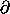1 H)i = 0.19 According to Hamilton's equations, the conjugate momentum pi is conserved. Hamilton's equations of motion for the remaining 2n - 2 phase-space variables do not involve qi (because it does not appear in the Hamiltonian), and pi is a constant. Thus the dimension of the difficult part of the problem, the part that involves the solution of coupled ordinary differential equations, is reduced by two. The remaining equation governing the evolution of qi in general depends on all the other variables, but once the reduced problem has been solved, the equation of motion for qi can be written so as to give Dqi explicitly as a function of time. We can then find qi as a definite integral of this function.20

Contrast this result with analogous results for more general systems of differential equations. There are two independent situations. One situation is that we know a constant of the motion. In general, constants of the motion can be used to reduce by one the dimension of the unsolved part of the problem. To see this, let the system of equations be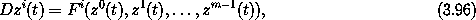where m is the dimension of the system. Assume we know some constant of the motion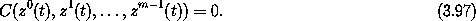At least locally, we expect that we can use this equation to solve for zm-1(t) in terms of all the other variables, and use this solution to eliminate the dependence on zm-1(t). The first m - 1 equations then depend only upon the first m - 1 variables. The dimension of the system of equations to be solved is reduced by one. After the solution for the other variables has been found, zm-1(t) can be found using the constant of the motion.

The second situation is that one of the variables, say zi, does not appear in the equations of motion (but there is an equation for Dzi). In this case the equations for the other variables form an independent set of equations of one dimension less than the original system. After these are solved, then the remaining equation for zi can be solved by definite integration.

In both situations the dimension of the system of coupled equations is reduced by one. Hamilton's equations are different in that these two situations often come together. If a Hamiltonian for a system does not depend on a particular coordinate, then the equations of motion for the other coordinates and momenta do not depend on that coordinate. Furthermore, the momentum conjugate to that coordinate is a constant of the motion. An added benefit is that the use of this constant of the motion to reduce the dimension of the remaining equations is automatic in the Hamiltonian formulation. The conserved momentum is a state variable and just a parameter in the remaining equations.

So if there is a continuous symmetry it will probably be to our advantage to choose a coordinate system that explicitly incorporates the symmetry, making the Hamiltonian independent of a coordinate. Then the dimension of the phase space of the coupled system will be reduced by two for every coordinate that does not appear in the Hamiltonian.21

#### Motion in a central potential

Consider the motion of a particle of mass m in a central potential. A natural choice for generalized coordinates that reflects the symmetry is polar coordinates. A Lagrangian is (equation 1.67):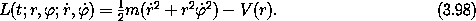The momenta are pr = mand p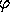= m r2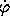. The kinetic energy is a homogeneous quadratic form in the velocities, so the Hamiltonian is T + V with the velocities rewritten in terms of the momenta: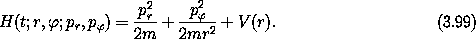Hamilton's equations are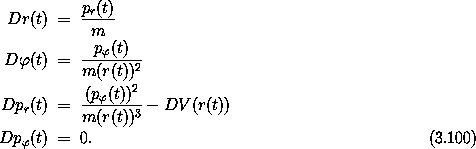The potential energy depends on the distance from the origin, r, as does the kinetic energy in polar coordinates, but neither the potential energy nor the kinetic energy depends on the polar angle. The angledoes not appear in the Lagrangian so we know that p, the momentum conjugate to, is conserved along realizable trajectories. The fact that pis constant along realizable paths is expressed by one of Hamilton's equations. That phas a constant value is immediately made use of in the other Hamilton's equations: the remaining equations are a self-contained subsystem with constant p. To make a lower-dimensional subsystem in the Lagrangian formulation we have to use each conserved momentum to eliminate one of the other state variables, as we did for the axisymmetric top (see section 2.10).

We can check our derivations with the computer. A procedure implementing the Lagrangian has already been introduced (below equation 1.67). We can use this to get the Hamiltonian:

(show-expression
((Lagrangian->Hamiltonian
(L-central-polar 'm (literal-function 'V)))
(up 't (up 'r 'phi) (down 'p_r 'p_phi))))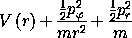and to develop Hamilton's equations:

(show-expression
(((Hamilton-equations
(Lagrangian->Hamiltonian
(L-central-polar 'm (literal-function 'V))))
(up (literal-function 'r)
(literal-function 'phi))
(down (literal-function 'p_r)
(literal-function 'p_phi)))
't))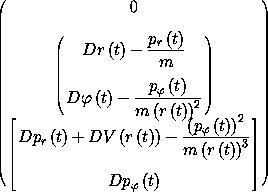#### Axisymmetric top

We reconsider the axisymmetric top (see section 2.10) from the Hamiltonian point of view. Recall that a top is a rotating rigid body, one point of which is fixed in space. The center of mass is not at the fixed point, and there is a uniform gravitational field. An axisymmetric top is a top with an axis of symmetry. We consider here an axisymmetric top with the fixed point on the symmetry axis.

The axisymmetric top has two continuous symmetries we would like to exploit. It has the symmetry that neither the kinetic nor potential energy is sensitive to the orientation of the top about the symmetry axis. The kinetic and potential energy are also insensitive to a rotation of the physical system about the vertical axis, because the gravitational field is uniform. We take advantage of these symmetries by choosing coordinates that naturally express them. We already have an appropriate coordinate system that does the job -- the Euler angles. We choose the reference orientation of the top so that the symmetry axis is vertical. The first Euler angle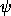expresses a rotation about the symmetry axis. The next Euler angle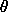is the tilt of the symmetry axis of the top from the vertical. The third Euler angleexpresses a rotation of the top about the fixed z axis. The symmetries of the problem imply that the first and third Euler angles do not appear in the Hamiltonian. As a consequence the momenta conjugate to these angles are conserved quantities. The problem of determining the motion of the axisymmetric top is reduced to the problem of determining the evolution ofand p. Let's work out the details.

In terms of Euler angles, a Lagrangian for the axisymmetric top is (see section 2.10):

(define ((L-axisymmetric-top A C gMR) local)
(let ((q (coordinate local))
(qdot (velocity local)))
(let ((theta (ref q 0))
(phidot (ref qdot 1))
(psidot (ref qdot 2)))
(+ (* 1/2 A
(square (* phidot (sin theta)))))
(* 1/2 C
(square (+ psidot (* phidot (cos theta)))))
(* -1 gMR (cos theta))))))

where gMR is the product of the gravitational acceleration, the mass of the top, and the distance from the point of support to the center of mass. The Hamiltonian is nicer than we have a right to expect:

(show-expression
((Lagrangian->Hamiltonian (L-axisymmetric-top 'A 'C 'gMR))
(up 't
(up 'theta 'phi 'psi)
(down 'p_theta 'p_phi 'p_psi))))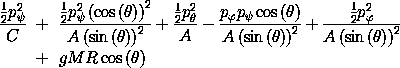Note that the anglesanddo not appear in the Hamiltonian, as expected. Thus the momenta pand pare constants of the motion.

For given values of pand pwe must determine the evolution ofand p. The effective Hamiltonian forand phas one degree of freedom, and does not involve the time. Thus the value of the Hamiltonian is conserved along realizable trajectories. So the trajectories ofand ptrace contours of the effective Hamiltonian. This gives us a big picture of the possible types of motion and their relationship, for given values of pand p.

If the top is standing vertically then p= p. Let's concentrate on the case that p= p, and define p = p= p. The effective Hamiltonian becomes (after a little trigonometric simplification)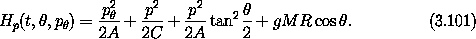Defining the effective potential energy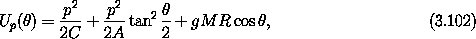which parametrically depends on p, the effective Hamiltonian is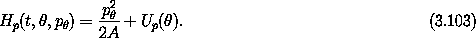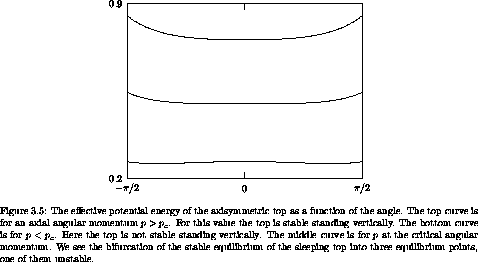If p is large, Up has a single minimum at= 0, as seen in figure 3.5. For small p there is a minimum for finite positiveand a symmetrical minimum for negative; there is a local maximum at= 0. There is a critical value of p at which= 0 changes from a minimum to a local maximum. Denote the critical value by pc. A simple calculation shows pc = (4 g M R A)1/2. For= 0 we have p = C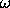, whereis the rotation rate. Thus to pc there corresponds a critical rotation rate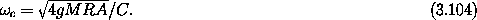For>c the top can stand vertically; for<c the top falls if slightly displaced from the vertical. The top that stands vertically is called the ``sleeping'' top. For a more realistic top, friction gradually slows the rotation; the rotation rate eventually falls below the critical rotation rate and the top ``wakes up.''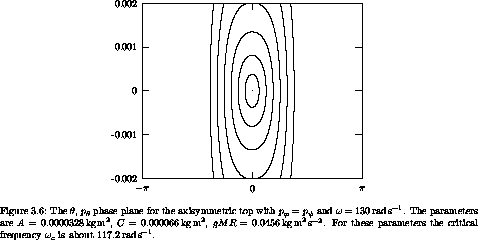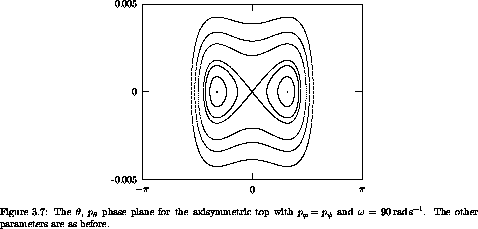We get additional insight into the sleeping top and the awake top by looking at the trajectories in the, pphase plane. The trajectories in this plane are simply contours of the Hamiltonian, because the Hamiltonian is conserved. Figure 3.6 shows a phase portrait for>c. All of the trajectories are loops around the vertical (= 0). Displacing the top slightly from the vertical simply places the top on a nearby loop, so the top stays nearly vertical. Figure 3.7 shows the phase portrait for<c. Here the vertical position is an unstable equilibrium. The trajectories that approach the vertical are asymptotic -- they take an infinite amount of time to reach it, just as a pendulum with just the right initial conditions can approach the vertical but never reach it. If the top is displaced slightly from the vertical then the trajectories loop around another center with nonzero. A top started at the center point of the loop stays there, and one started near this equilibrium point loops stably around it. Thus we see that when the top ``wakes up'' the vertical is unstable, but the top does not fall to the ground. Rather, it oscillates around a new equilibrium.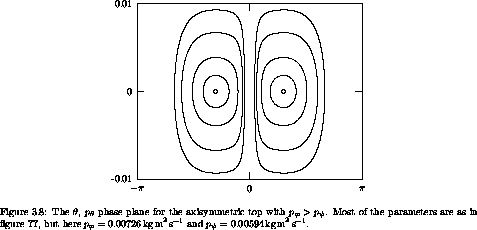It is also interesting to consider the axisymmetric top when pne p. Consider the case p> p. Some trajectories in the, pplane are shown in figure 3.8. Note that in this case trajectories do not go through= 0. The phase portrait for p< pis similar and is not shown.

We have reduced the motion of the axisymmetric top to quadratures by choosing coordinates that express the symmetries. It turns out that the resulting integrals can be expressed in terms of elliptic functions. Thus, the axisymmetric top can be solved analytically. We do not dwell on this solution because it is not very illuminating: since most problems cannot be solved analytically, there is little profit in dwelling on the analytic solution of one of the rare problems that is analytically solvable. Rather, we have focused on the geometry of the solutions in the phase space and the use of integrals to reduce the dimension of the problem. With the phase-space portrait we have found some interesting qualitative features of the motion of the top.

Exercise 3.8.  Sleeping top
Verify that the critical angular velocity above which an axisymmetric top can sleep is given by equation (3.104).

### 3.4.1  Lagrangian Reduction

Suppose there are cyclic coordinates. In the Hamiltonian formulation, the equations of motion for the coordinates and momenta for the other degrees of freedom form a self-contained subsystem in which the momenta conjugate to the cyclic coordinates are parameters. We can form a Lagrangian for this subsystem by performing a Legendre transform of the reduced Hamiltonian. Alternatively, we can start with the full Lagrangian and perform a Legendre transform for only those coordinates that are cyclic. The equations of motion are Hamilton's equations for those variables that are transformed and Lagrange's equations for the others. The momenta conjugate to the cyclic coordinates are conserved and can be treated as parameters in the Lagrangian for the remaining coordinates.

Divide the tuple q of coordinates into two subtuples q = (x, y). Assume L(t; x, y; vx, vy) is a Lagrangian for the system. Define the Routhian R as the Legendre transform of L with respect to the vy slot: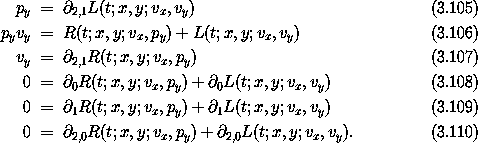To define the function R we must solve equation (3.105) for vy in terms of the other variables, and substitute this into equation (3.105).

Define the state path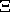: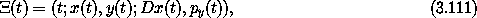whereRealizable paths satisfy the equations of motion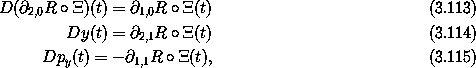which are Lagrange's equations for x and Hamilton's equations for y and py.

Now suppose that the Lagrangian is cyclic in y. Then1,1 L =1,1 R = 0, and py(t) is a constant c on any realizable path. Equation (3.113) does not depend on y, by assumption, and we can replace py by its constant value c. So equation (3.113) forms a closed subsystem for the path x. The Lagrangian Lc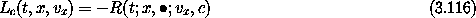describes the motion of the subsystem (the minus sign is introduced for convenience, and ¤ indicates that the function's value is independent of this argument). The path y can be found by integrating equation (3.114) using the independently determined path x.

Define the action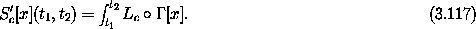The realizable paths x satisfy the Lagrange equations with the Lagrangian Lc, so the action S'c is stationary with respect to variations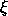of x that are zero at the end times: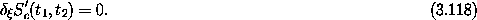For realizable paths q the action S[q](t1, t2) is stationary with respect to variations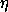of q that are zero at the end times. Along these paths the momentum py(t) has the constant value c. For these same paths the action S'c[x](t1, t2) is stationary with respect to variationsof x that are zero at the end times. The dimension ofis smaller than the dimension of.

The values of the actions S'c[x](t1, t2) and S[q](t1, t2) are related: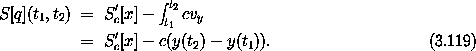Exercise 3.9.  Routhian equations of motion
Verify that the equations of motion are given by equations (3.113-3.115).

19 If a Lagrangian does not depend on a particular coordinate then neither does the corresponding Hamiltonian, because the coordinate is a passive variable in the Legendre transform. Such a Hamiltonian is said to be cyclic in that coordinate.

20 Traditionally, when a problem has been reduced to the evaluation of a definite integral it is said to be reduced to a ``quadrature.'' Thus, the determination of the evolution of a cyclic coordinate qi is reduced to a problem of quadrature.

21 It is not always possible to choose a set of generalized coordinates in which all symmetries are simultaneously manifest. For these systems, the reduction of the phase space is more complicated. We have already encountered such a problem: the motion of a free rigid body. The system is invariant under rotation about any axis, yet no single coordinate system can reflect this symmetry. Nevertheless, we have already found that the dynamics is described by a system of lower dimension that the full phase space: the Euler equations.